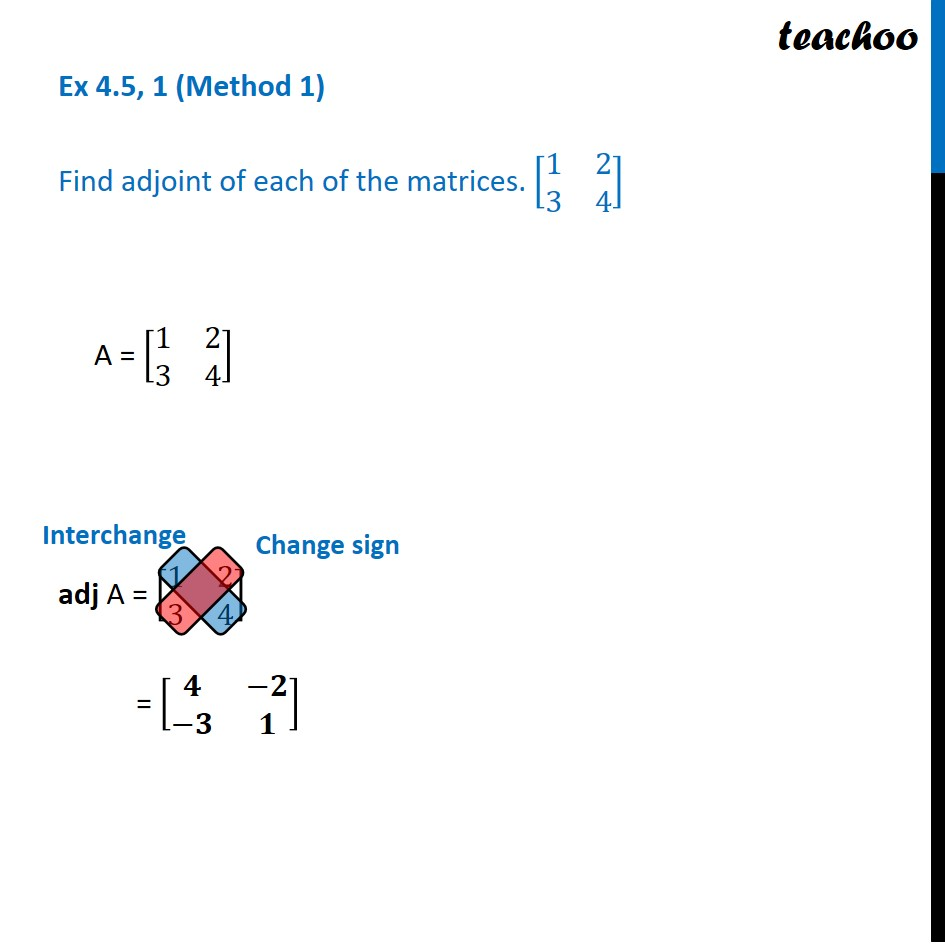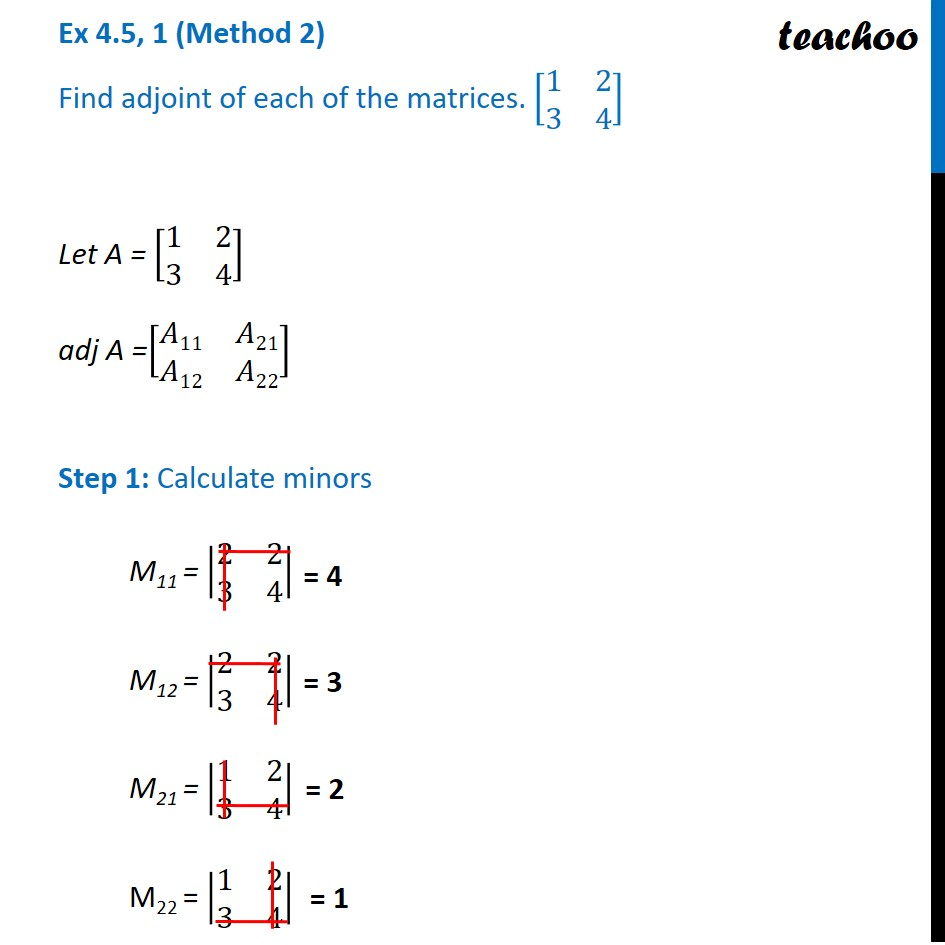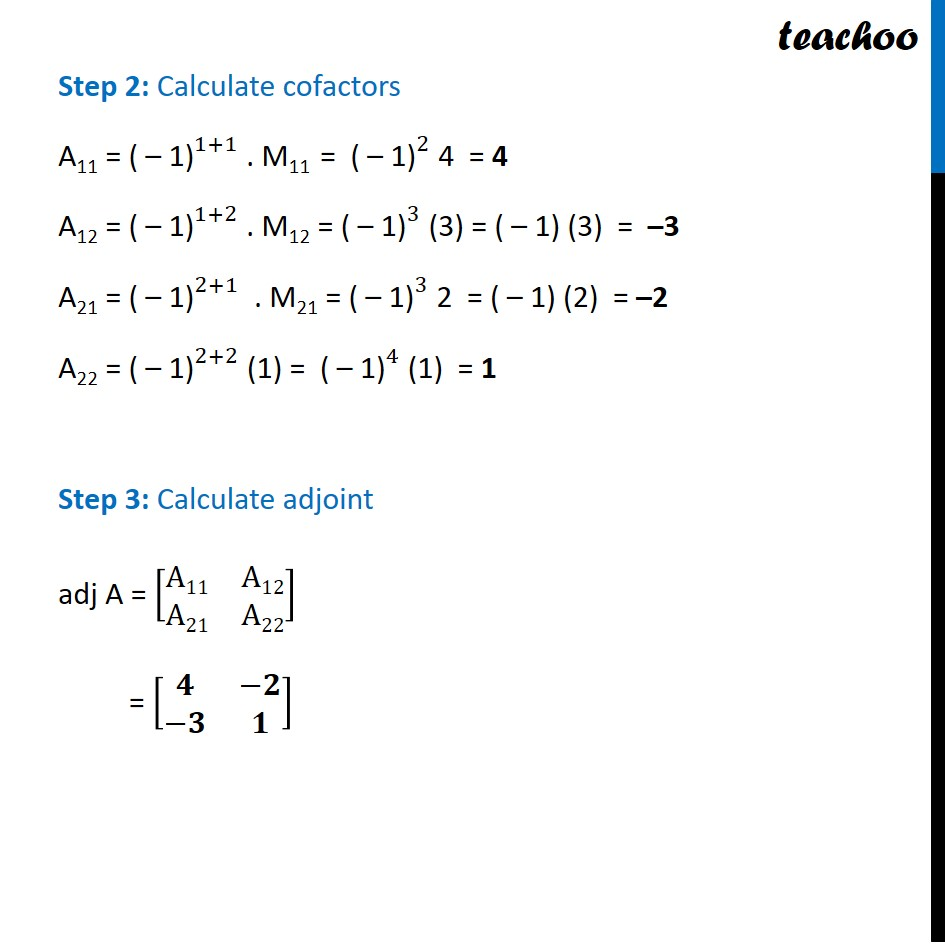Ex 4.4

Chapter 4 Class 12 Determinants
Serial order wiseLearn in your speed, with individual attention - Teachoo Maths 1-on-1 Class

### Transcript

Ex 4.4, 1 (Method 1) Find adjoint of each of the matrices. [■8(1&[email protected]&4)] A = [■8(1&[email protected]&4)] adj A = [■8(1&[email protected]&4)] = [■8(𝟒&−𝟐@−𝟑&𝟏)] Ex 4.4, 1 (Method 2) Find adjoint of each of the matrices. [■8(1&[email protected]&4)] Let A = [■8(1&[email protected]&4)] adj A =[■8(𝐴11&𝐴21@𝐴12&𝐴22)] Step 1: Calculate minors M11 = |■8(2&[email protected]&4)| M12 = |■8(2&[email protected]&4)| M21 = |■8(1&[email protected]&4)| M22 = |■8(1&[email protected]&4)|Step 2: Calculate cofactors A11 = 〖"( – 1)" 〗^(1+1) . M11 = 〖"( – 1)" 〗^2 4 = 4 A12 = 〖"( – 1)" 〗^(1+2) . M12 = 〖"( – 1)" 〗^3 (3) = ( – 1) (3) = –3 A21 = 〖"( – 1)" 〗^(2+1) . M21 = 〖"( – 1)" 〗^3 2 = ( – 1) (2) = –2 A22 = 〖"( – 1)" 〗^(2+2) (1) = 〖"( – 1)" 〗^4 (1) = 1 Step 3: Calculate adjoint adj A = [■8(A11&[email protected]&A22)] = [■8(𝟒&−𝟐@−𝟑&𝟏)]